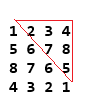### Find sum of all elements above diagonal in matrix - program in java

Write a program to Find sum of all elements above diagonal in matrix in java.Sum of elements above diagonal = 2+3+4+7+8+5= 29

Logic behind finding sum of elements above diagonal is:
//Logic to calculate sum of elements above diagonal.
int sum=0;
for (int j = 1; j < columns; j++) {
for (int i=j-1 ; i>=0 ; i--) {
sum= sum + matrix[i][j];
}

}

Example/ Full Program/SourceCode to Find sum of elements above diagonal in matrix in java >
 package matrix; import java.util.Scanner; /** Copyright (c), AnkitMittal  JavaMadeSoEasy.com Find sum of elements above diagonal in matrix in java*/ public class SumOfElementsAboveDiagonal {    public static void main(String...args) {                     Scanner scanner = new Scanner(System.in);           System.out.print("Enter number of rows/columns in matrix : ");    //rows and columns in matrix must be same.           int rows = scanner.nextInt();           int columns=rows;           int[][] matrix = new int[rows][rows];                     System.out.println("Enter the elements in matrix :");           for (int i = 0; i < rows; i++) {                  for (int j = 0; j < columns; j++) {                        matrix[i][j] = scanner.nextInt();                  }           }           //Logic to calculate sum of elements above diagonal.           int sum=0;           for (int j = 1; j < columns; j++) {                  for (int i=j-1 ; i>=0 ; i--) {                        sum= sum + matrix[i][j];                  }                            }           System.out.println("\nMatrix is : ");           for (int i = 0; i < rows; i++) {                  for (int j = 0; j < columns; j++) {                        System.out.print(matrix[i][j] + " ");                  }                  System.out.println();           }           System.out.println("sum of elements above diagonal is: "+sum);    } } /*OUTPUT Enter number of rows/columns in matrix : 4 Enter the elements in matrix : 1 2 3 4 5 6 7 8 8 7 6 5 4 3 2 1 Matrix is : 1 2 3 4 5 6 7 8 8 7 6 5 4 3 2 1 sum of elements above diagonal is: 29   */

We Wrote a program to Find sum of all elements above diagonal in matrix in java.

Having any doubt? or you you liked the tutorial! Please comment in below section.